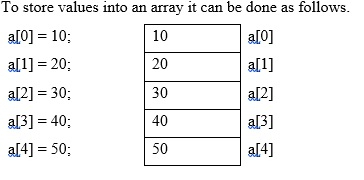# Write a C program to reverse array

An array is a group of related items that store with a common name.

## Syntax

The syntax is as follows for declaring an array −

datatype array_name [size];

## InitializationAn array can also be initialized at the time of declaration −

int a = { 10,20,30,40,50};

## Reversing array in C

We can reverse array by using swapping technique.

For example, if 'P' is an array of integers with four elements −

P = 1, P = 2, P = 3 and P=4

Then, after reversing −

P = 4, P = 3, P = 2 and P=1

## Example

Following is the C program to reverse an array −

#include <stdio.h>
int main(){
int num, i, j, array1, array2;
printf("Enter no of elements in array");
scanf("%d", &num);
printf("Enter array elements");
for (i = 0; i < num ; i++)
scanf("%d", &array1[i]);
// Copying elements into array
for (i = num - 1, j = 0; i >= 0; i--,j++)
array2[j] = array1[i];
// Copying reversed array into the original
for (i = 0; i < num; i++)
array1[i] = array2[i];
printf("The reversed array:");
for (i = 0; i< num; i++)
printf("%d", array1[i]);
return 0;
}

## Output

Upon execution, you will receive the following output −

Enter no of elements in array
4
Enter array elements
20
50
60
70
The reversed array:
70
60
50
20